Marton Veges 2022-05-16T22:00:00+02:00 https://mveg.es Marton Veges mail@mveg.es Inside Windows Registry 2020-06-16T22:00:00+02:00 https://mveg.es/posts/inside-windows-registry/ The Windows Registry is a central storage for settings in Windows, and also a popular target of hacks to enable/disable obscure features. But how does it work? Where does it store all the data? What options are available in it? In this post I plan to go through the internals of the registry.

## Overview

The registry is a hierarchical key-value storage system, that keeps its elements in a tree-like structure, much like a file system. The values have data types so not only strings but integers and binary data can be stored as well.

The "folders" in the registry are called keys. This is unlike other key-value stores, like Redis, where key refers to the id of a value. The actual key-value pairs inside these folders are called name-data pairs. For example, HKLM\SOFTWARE\Microsoft\Windows\CurrentVersion is a key, and the name ProgramFilesDir under this key holds the path to the "Program Files" folder. This strange naming has historical reasons: in the original version of the registry (in Windows 3.1) keys could store only a single value. This naming scheme was kept for backward-compatibility. It is also the reason why each key has a 'default' value.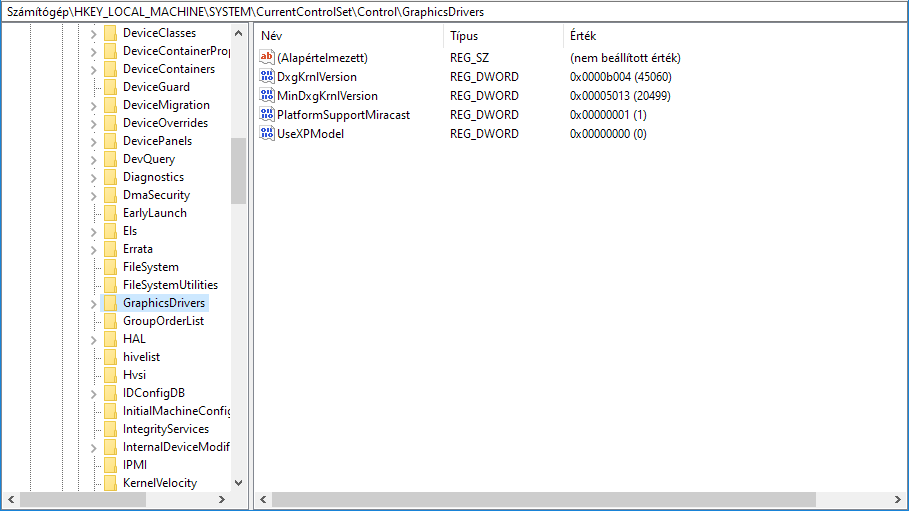## Data types

One advantage of the registry over simple ini files is that it can store typed data, and possibly binary data. Some of the more important datatypes:

ID Symbolic Name Description
0 REG_NONE No type
1 REG_SZ A string
2 REG_EXPAND_SZ An expandable string: can contain environment variables that are substituted
3 REG_BINRAY Binary data
4 REG_DWORD A 32 bit integer (double word)
6 REG_LINK Symbolic link to another registry key
7 REG_MULTI_SZ An array of strings, strings are separated by the NUL character
11 REG_QWORD A 64-bit integer (quad word)

There are other types available, the full list is available here.

## Hives

The registry is not stored as a single file on disk but as multiple files, each representing one subtree of the full registry. For example, HKEY_CURRENT_USER is stored in %user_home%\ntuser.dat. These files are called hives. However, hives and root keys do not have a one-to-one correspondence. Check the next section on how each root key is actually stored.

You can list the available hives in the registry itself: they are stored under HKEY_LOCAL_MACHINE\SYSTEM\CurrentControlSet\Control\hivelist. It lists all the hives with the locations they are mounted at.The hive files use a binary dataformat. The basic element in the file is the cell. A cell can be a key, a value (with name and data), a list of keys or a list of values. Then a key cell points to a list-of-keys cell to represent its subkeys and to a list-of-values cell to represent the values under the key.

Windows also keep transaction logs for each hive. They have the same name as the hive, with an additional .log1 or .log2 suffix. These files ensure that if the computer crashes while the registry is edited, no data is lost. Why are there two log files? If the system stops while .log1 is written, the new data will be added to .log2. This serves as a double protection mechanism against crashes.

## Root keys

Root keys are the top level keys in the database, their name all start with HKEY. In general, HKEY_LOCAL_MACHINE keeps the computer level settings, while HKEY_CURRENT_USER has the settings for the current user. When a program wants to access a certain setting it should first look into HKEY_CURRENT_USER then search HKEY_LOCAL_MACHINE for the key.

### HKEY_LOCAL_MACHINE

This key stores the computer level settings that are not specific to any users. The SAM, SECURITY, SOFTWARE and SYSTEM subkeys are stored in the folder %windir%\system32\config, each in a different file. The HARDWARE key is not stored on disk: it is generated during startup and kept entirely in memory. The BCD00000000 key is a virtual mapping of the BCD database (Boot Configuration Data, where the bootloader keeps the information on the available operating systems).

The applications should place their settings in the SOFTWARE subkey, under a key named Vendor\ApplicationName.

### HKEY_USERS

The user specific settings. Users are referred by the Security Identifiers (SID), it is a long string looking something like S-15-20-.... Usually only the current user is loaded, plus some additional virtual users used for LAN file sharing and SharePoint. Each user has two subkeys: one that is named after the SID of the user, and another that has _CLASSES appended to it. The latter contains information about registered applications, file types and OLE class IDs. The other contains all the additional user-level settings. Similarly to HKEY_LOCAL_MACHINE, programs should store their settings in Software\Vendor\ApplicationName. The keys are stored in the %user_home%\ntuser.dat and %user_home%\AppData\Local\Microsoft\Windows\usrclass.dat hives.

### HKEY_CURRENT_USER

This is simply a pointer to the current user's key in HKEY_USERS.

### HKEY_CLASSES_ROOT

This key contains all information about file associations and registered COM components. While it is not stored directly on disk, it is neither a simple pointer to another key. Instead it merges the user level component information stored in HKEY_USER\current_user_sid_CLASSES and the system level information in HKLM\Software\Classes. If a key occurs in both places, the user-level value takes precedence.

### HKEY_CURRENT_CONFIG

Contains information used by Windows during startup. It is a Pointer to HKEY_LOCAL_MACHINE\System\CurrentControlSet\Hardware Profiles\Current.

]]>
Linux Server on an Android Phone 2021-01-10T23:00:00+01:00 https://mveg.es/posts/linux-server-on-an-android-phone/ A little while ago I wanted to set up a small home server to host some pages. I could have just used some free web hosting but where is the fun in that? So I checked what old devices I have I could repurpose. The first obvious choice was my old HP laptop. It had a tiny bit of a problem: its fans are super loud, I didn't want to listen to them all day.

Then it downed on me: I just bought a new Android phone in November, my previous Sony Z3C can serve as the server! Smartphones of today have way more power than servers in the 90s so it should be able to handle the task, and I might also set up PHP for Wordpress.

There was only a single problem: the touchscreen is broken and doesn't register half of the taps on the screen. It turns out this is an easy problem to solve: scrcpy lets you view the screen of your phone on your computer and also simulates clicks. What is even better, you can use your laptop keyboard to simulate a hardware keyboard for the phone. This will come immensely useful later, as we will have to type quite a few commands.

To use scrcpy, you need to enable USB debugging on the phone. Luckily, I'm an android developer and it was already turned on. Otherwise I would have a hard time setting it, since the touchscreen doesn't work properly.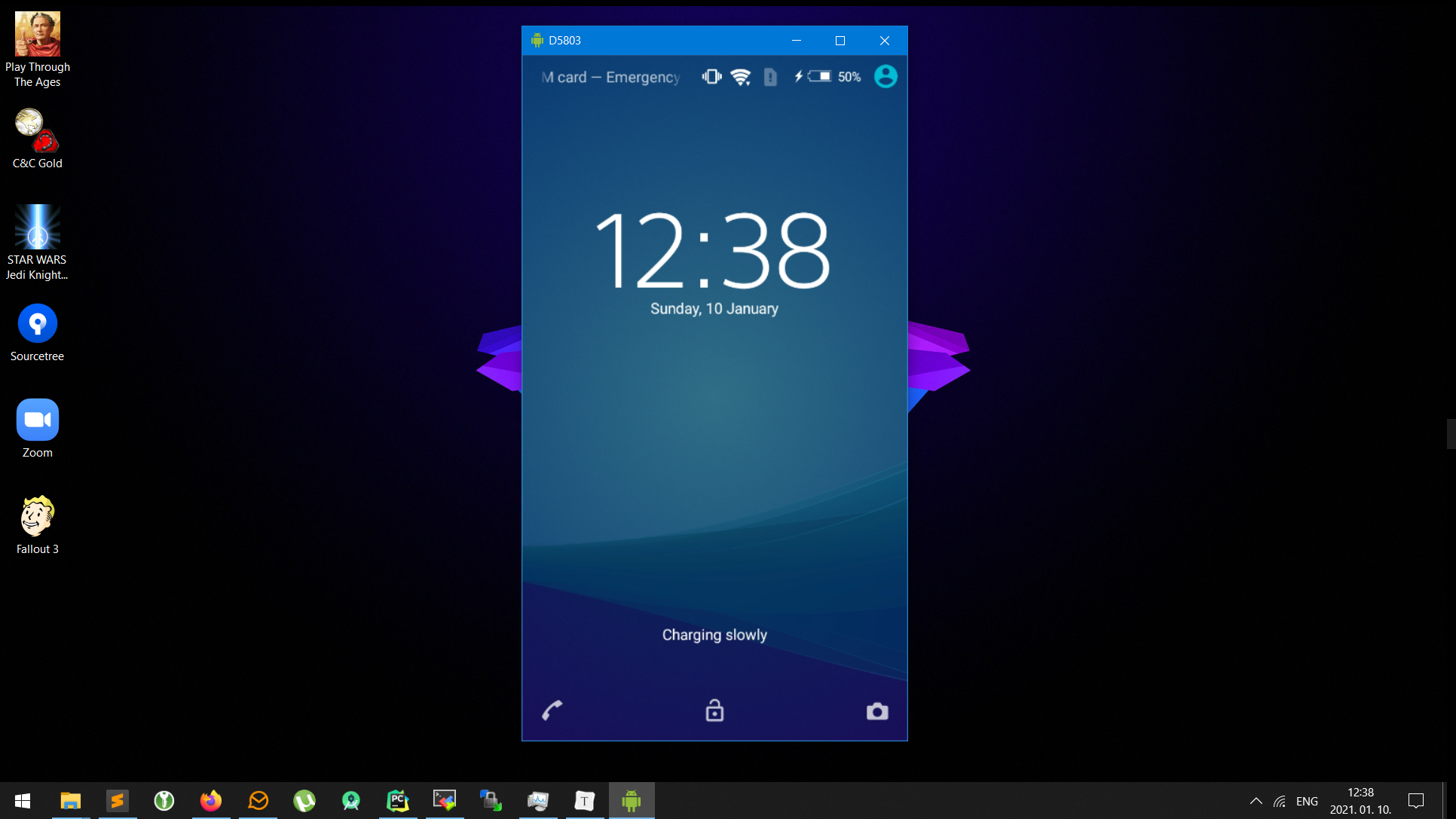## Setting up "Ubuntu"

So I can control the device again! Next step is to set up the server. I wanted to make the phone more than a simple webserver, for that I could have downloaded an app. I wanted it to have SSH and the ability to run any (ok, most) Linux programs. After a bit of research there seemed to be two ways: either with or without rooting. I did not want to root the phone as it causes a factory reset, and I'm afraid I won't be able to turn the developer options on again.

So, no-root solutions remain. The basis of the setup is Termux. It is an amazing project: they made a fully functional terminal emulator for Android. What is amazing, they have a repository of packages that were all patched and rebuilt for Android. I could have stopped here, they have Apache, PHP, python, all the goodies I needed. But why not extend the set of available packages to all ARM Linux packages?Android is very far from a real Linux OS, especially if you don't have root. The biggest problem is the file system: there are no /usr,/var,/proc folders, the standard libc library is different,…. To be able to run packages built for Ubuntu, one has to fix all of these, plus library loading paths, etc. If you have root access, you can call chroot and set up a contained file system, it is a good starting point. Unfortunately, I do not have root.

Here comes proot into the picture: it is like chroot, without the need of root access. Basically, it runs your application via ptrace and dynamically replaces system calls. I wonder if it has any performance implications but I haven't done any tests yet.

Having proot, we can easily simulate a filesystem, change the user to root, setup a home dir. I chose the easier path and didn't do it all by myself, instead used the Andronix Ubuntu distributions. It needs a single command executed in Termux, and takes care everything itself. When finished, you have a fully simulated Ubuntu on your phone! It can run even X11 apps though I haven't tried it. You can run apt get to install packages like you would do on a real computer.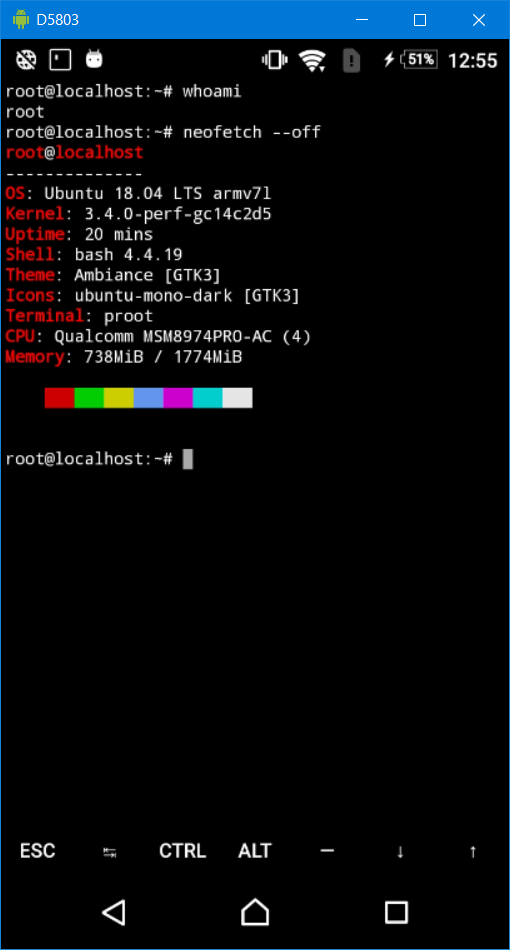## No dozing please

One thing that was left to do: disabling android battery optimization on Termux. By default, Android throttles background apps and that's not what you want from your server. Fortunately, this is a single checkbox in the Settings menu.

## Final steps

After everything set up, let's try the simple HTTP server from python:

> python3 -m http.server 8000

And the results: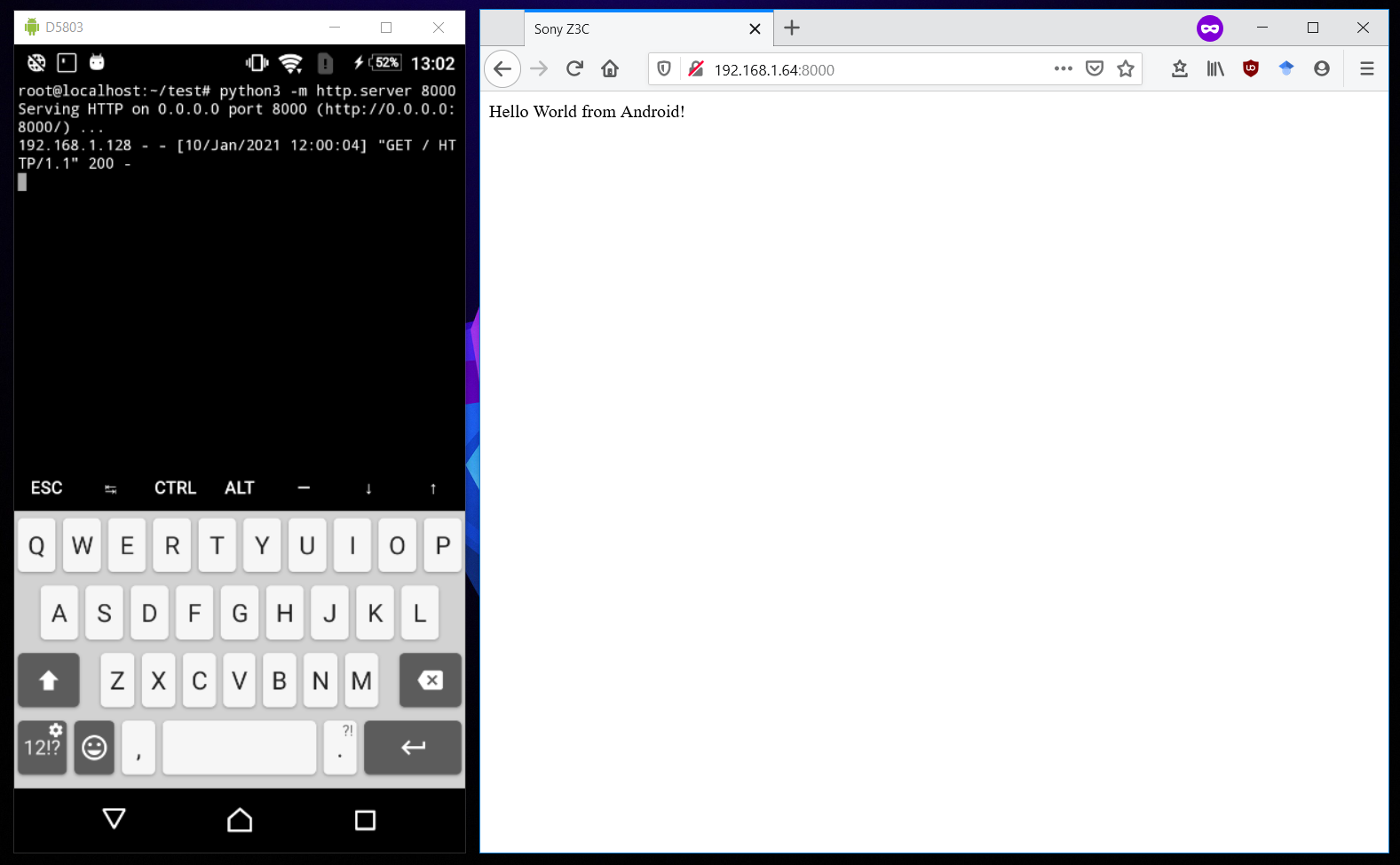]]>
Writing a device driver for Unix V6 2022-03-08T23:00:00+01:00 https://mveg.es/posts/writing-a-device-driver-for-unix-v6/ In this post we will learn the useful skill of writing a device driver for Unix V6 (released in 1975) and run it on an emulator. The implemented device is fairly trivial: it will open a message box on the host OS. The goal is that we can execute the following command:
echo "Hello world" > /dev/mb
And get this: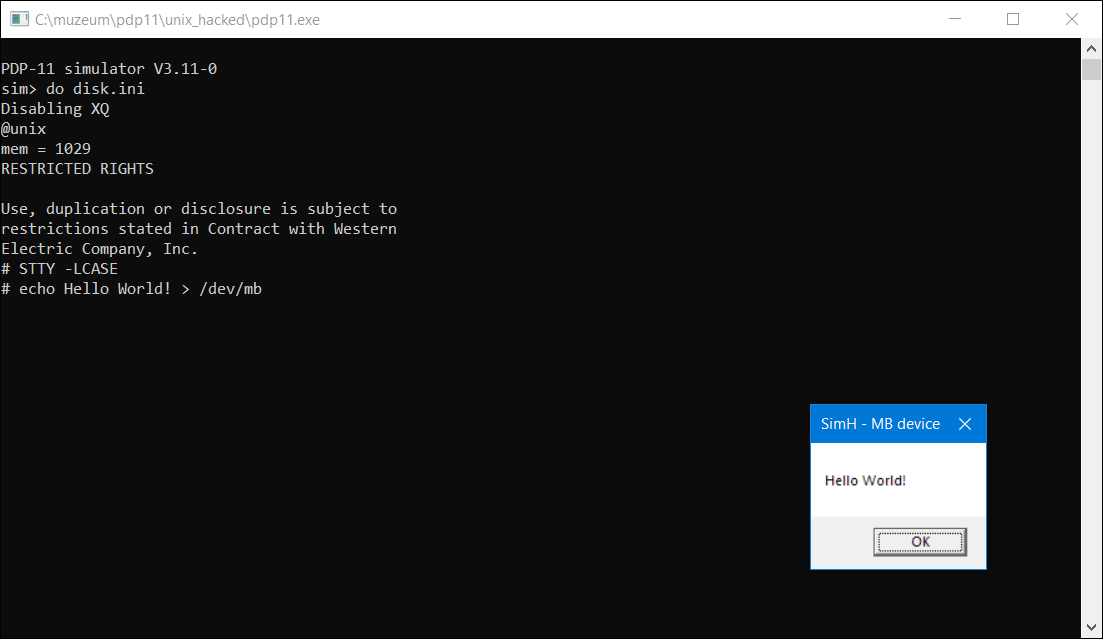The message box is opened in the host OS, so effectively we create a special file (/dev/mb) that accepts text input and opens a message box.

Since Unix V6 runs on PDP-11 and I do not have access to one, everything is run via SimH. SimH is a family of old computer emulators, ranging from IBM 1401 to Altair 8800. We only need the PDP-11 one though.

## Background

Unix V6 was developed in 1975 on a PDP-11. By this version, most of the code was written in C and only a couple of hundreds assembly code remained. Already, it had many of the utilities that are common on a today's Linux system: cp, rm, bc/dc, cron, dd, chmod, ls. The OS was very powerful: had memory management, multiple users and pre-emptive multitasking. It is funny to see that many of these features arrived only in the 90s for personal comptuters, while bigger minicomputers had them for decades.

The choice of Unix V6 is motivated by two facts: first, it is written mostly in C, thus easy to understand. Second, there exists a full documentation of the kernel code. John Lion's Commentary on UNIX 6th Edition is a thorough description of the inner workings of Unix and the underlying algorithms. It is begginer friendly, guiding you through the kernel step-by-step.

PDP-11 was a family of computers produced between 1970 and 1990. The Bell Research Lab used these devices so both the Unix OS and the C language was developed primarily on them. PDP-11 minicomputers introduced inportant innovations, that let Thompson and Ritchie implement several advanced features for Unix: hardware support for memory paging, user and kernel mode, different priority levels for interrupts. Again, CPUs of personal computers only had these in the late 80s – early 90s.

## Implementing a new device

To add the new device to Unix, first we have to create a device in the emulator. On the PDP-11 it was common to map certain regions of the memory to a device and communicate with the device via this memory region. When a value was written to a particular address, the values were forwarded to the device. Conversely, the status of the device could be read by reading the right locations.

We will follow the same strategy. The device, called mb, is mapped to the 17777340-17777342 addresses (addresses are in octal). The characters of the message are written one-by-one at 17777340 which our virtual device reads and stores the letters in a buffer. Upon receiving a newline or zero (\0) character the buffer is converted to a string and displayed in a message box. The device handles ASCII character only, however PDP-11 has a 16 bit CPU, so 2 bytes are written at a time. This is the reason why we have mapped a 2 bytes long region instead of a single byte.

Our choice of emulator is SimH. SimH is not actually an emulator but a framework for writing emulators. Roughly speaking, it lets you define devices that can be attached to an emulated machine. These devices include peripherals such as magnetic tape reader, disk drive or teletypes. When attached, a device provides additional registers and can be memory-mapped to a hardcoded region. The implementation interface is quite simple: a pair of read/write functions must be defined for the registers and the memory-mapping. Since want to use only the latter, and only for output, it is enough to implement the memory write function:

#define BUF_LEN 250TCHAR buf[BUF_LEN+1];int cnt;t_stat mb_wr (int32 data, int32 PA, int32 access){	char c = data & 0xff;	if (c == '\n' || c == 0 || cnt >= BUF_LEN) {	    display_message();	    mb_reset(NULL);	}	else {	    buf[cnt++] = c;	}	return SCPE_OK;}void display_message(){    MessageBox(        NULL,        (LPCTSTR)buf,        (LPCTSTR)L"SimH - MB device",        0    );}

The function takes an address (PA) and a value (data) that will be written to the address. Since our virtual device supports ASCII only, we take the lower byte. If the buffer is full or we have received a newline or \0 character, the message box is shown. Otherwise, the character is appended to the buffer. We ignore the address, as the device is attached to a single word address anyway.

All that's left is to connect the device to a certain memory address and tell SimH the name of the write function.

#define IOBA_MB         017777340           /* MB - message box */#define IOLN_MB         002DIB mb_dib = { IOBA_MB, IOLN_MB, NULL, &mb_wr, 0 }

In the mb_dib structure, the first value is the start of the memory segment, followed by the length and the memory read-write functions. The device does not support reading so the read function is NULL. The mb_dib structure is then passed to SimH to make it aware of the existence of mb.

Let's test if the new device works. After firing up SimH:

# Activating and initializing the devicesim> set mb enabled  sim> reset# write 'A\0' to the device by poking memorysim> deposit 17777340 101sim> deposit 17777340 0

First, the device have to be turned on an initialized with reset. Then, the octal values 101 and 0 are written at the adress 17777340. Upon writing the 0 byte, the message box appears: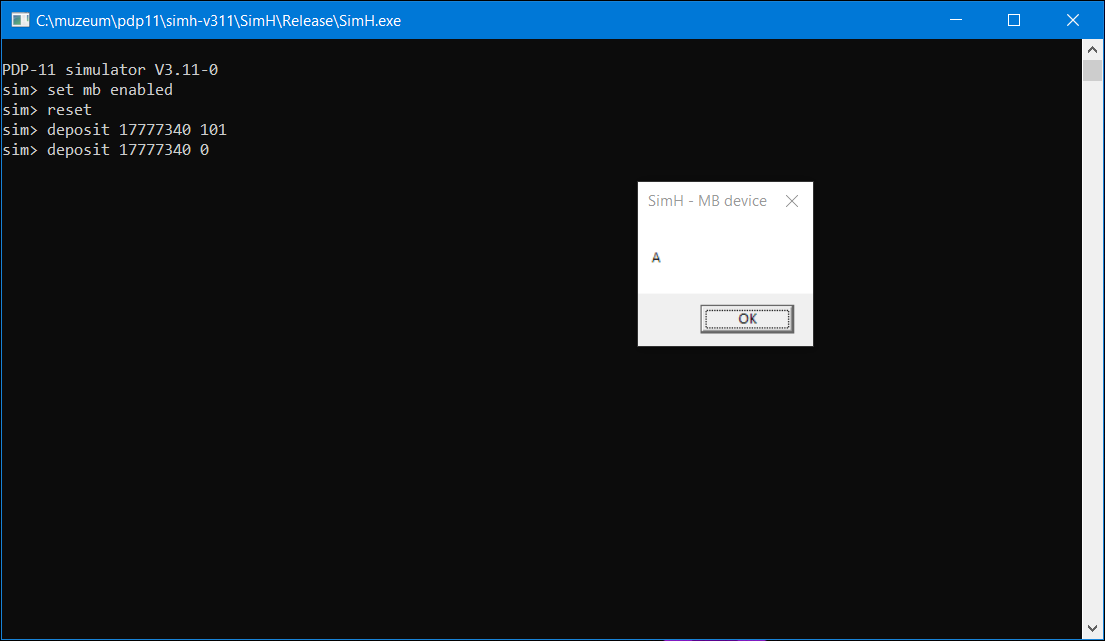## Implementing the driver

Let's move on to the implementation of the driver itself. In UNIX V6, there are two types of devices: block and character special devices. The first one uses a buffering mechanism implemented in the kernel and reads/writes blocks of data. Typically, floppy and cartridge drives use this method. Character devices can do whatever they want, though most of the time they will print/read one character at a time. Examples are terminals and paper tape punchers.

#define	MBADDR	0177340struct {	int mbchr;};mbwrite(){	register int c;	while ((c=cpass())>=0) {		MBADDR->mbchr = c;	}}

UNIX will call the mbwrite function when something is written to the device. mbwrite calls the cpass function in a loop, cpass returns the next character from the argument of the write system call. There is one strangeness in this code: the anonymous struct and using the MBADDR address as a struct. This is an ancient behaviour of the C language from the early 70s. Back then, struct field names were kind of global, meaning when seeing an expression var->structfield, the compiler ignored the type of var and calculated the offset based on structfield alone. This also meant that field names in structs had to be unique. In this code MBADDR->mbchr is equivalent to *(MBADDR+0). This saves putting MBADDR into a temporary pointer and dereferencing the pointer.

The second quirk astute readers might have noticed is that we are writing to the address 177340 while the device was mapped 17777340. This is because UNIX is using virtual memory to have access to more than the 64kb RAM that 16 bit would allow to address. In kernel mode, the last 8kb of virtual memory (160000 to 177777) is always mapped to the last 8kb of the physical memory (17760000 to 17777777 in the emulator configuration).

Having the device driver, we just have to tell the kernel about it by adding an entry to the device configuration table:

int     (*cdevsw[])(){        &klopen,   &klclose,  &klread,   &klwrite,  &klsgtty,   /* console */		...        &nulldev,   &nulldev,  &nodev,    &mbwrite,  &nodev,    /* mb */        0};

The table contains the implementations of the open, close, read and write system calls for every device. By the way, this table can be generated automatically by the mkconf utility.

After rebuilding the kernel, we can mount the device like this:

/etc/mknod /dev/mb c 16 0

The name of the mountpoint is /dev/mb, c means it is a character special device. 16 is the major device number, it is the row index of the driver in the cdevsw table. 0 is the minor device number: when multiple devices of the same type are attached, this signifies the id of the specific device.

Now let's see what happens, when someone enters the following code to the shell:

echo Hello world! > /dev/mb

The shell, after parsing the command, will call the write system call, which just calls rdwr. rdwr is the common implementation of the read and write system calls. After checking permissions, it decides whether the file is a pipe or inode, and eventually calls the writei function (link). writei means "write inode", that is it will write to that node in the file system. Since /dev/mb is a character special file, writei quickly dispatches the code to the driver:

if((ip->i_mode&IFMT) == IFCHR) {	(*cdevsw[ip->i_addr.d_major].d_write)(ip->i_addr);	return;}

The if clause checks if the inode to write to is a character device. If yes, it will look up the write function of the device in the cdevsw table (using the major device number as index) and call it with the minor device number (stored in ip->i_addr). The d_write function is mapped to mbwrite and will write the string one character at a time to the address 0177340. This triggers our device's code in the emulator and results in showing the message box.

]]>
Fast numerically stable moving average 2022-05-16T22:00:00+02:00 https://mveg.es/posts/fast-numerically-stable-moving-average/ Calculating the moving average of a time series is a pretty simple problem. Just loop over each position, and add up the elements over the window:

void simple_ma(double* in, double* out, int N, int W) {    for (int i = 0; i < N - W + 1; i++) {        double sum = 0;        for (int j = 0; j < W; j++) {            sum += in[i + j];        }        out[i] = sum / W;    }}

However, this has a time complexity of $O(NW)$ which means for a large $W$ (window size) it becomes quite slow. On my machine, with $N=1000 000$ and $W=1000$ the computation takes nearly a second. We can implement the summation with SIMD instructions, but it only gives us a speed up of a factor of 4 (on a typical AVX2 machine one can perform 4 double precision addition in a single instruction).

## Naive approach

There is a very simple trick to speed the calculation up and reach a time complexity of $O(N+W)$. First calculate the sum of the first $W$ elements, then add and subtract the newest and oldest elements when moving the window:

void summing_ma(double* in, double* out, int N, int W) {    double sum = 0;    for (int i = 0; i < W; i++) {        sum += in[i];    }    out = sum / W;    for (int i = W; i < N; i++) {        sum = sum + in[i] - in[i - W] ;        out[i - W + 1] = sum / W;    }}

This is fast, however fails miserably when your numbers are of different scale. Let's check its output for the following input:

double in[] = {1, 1, 1, 1e17, 1, 1, 1, 1};// Output1  3.333e+16  3.333e+16  3.333e+16     0     0

After the fifth element, the result becomes zero, reaching a relative error of 100%. What has happened? We just hit the main problem of floating point calculations: catastrophic cancellation.

To understand catastrophic cancellation, let's review how floating point numbers are represented. Floating point numbers can be written as $$s\times a\times 2^k,$$ where $s\in \{-1,1\}$ is the sign, $a$ is the significand and $k$ is the exponent. $a$ is normalized such that it is between 1 and 2. This way its first digit is always 1 and can be omitted in the binary representation.

Multiplying two floating point numbers is easy, just multiply the significand and sum the exponents: $$s_1s_2\times a_1a_2\times 2^{k_1+k_2}.$$ It doesn't matter if one of the numbers is small and the other is large, the significands can be still multiplied, resulting in no loss of precision. However, that's not the case with additions. If one of the addends is much smaller than the other, there is a loss of precision. Essentially, an addition can be written as (for simplicity, assume both numbers are positive and $k_1>k_2$): $$a_1\times 2^{k_1}+a_2\times 2^{k_2} = (a_1+a_22^{k_2-k_1})\times 2^{k_1}.$$ The exponent will be the larger of the two and the significands are added with the second one scaled. If $k_1$ is much larger than $k_2$, $a_2$ will be scaled down by several digits. Since we have a limited number of bits to represent the significand, only the highest bits of $a_2^{k_2-k_1}$ are added to $a_1$. The rest is simply cut off, resulting in lost precision.

In extreme cases, the sum might not change at all:

1e17 + 1 = 1e17

That is, adding 1 to 1e17 does not change the value. How does this relate to our buffered moving average? When adding 1e17 to the sum, the variable sum becomes 1e17. On the fifth iteration, when 1e17 leaves the window, this is what the implementation does:

(sum + 1) - 1e17 = (1e17 + 1) - 1e17 = 1e17 - 1e17 = 0

So the output for the fifth average is zero. To make matters worse, sum is corrupted now! That is, the average of every subsequent window will be zero, introducing large errors forever.

This is a pretty serious flaw of the algorithm. Imagine you are collecting measurements, 1 million timestamps, and you have an erroneous number somewhere. Maybe you do some preprocessing and a near zero value ends up as a very large number after a division. Our fast moving average algorithm will produce nonsensical results after the bad measurement, making all the output useless. That is, a single measurement error invalidates your million elements long time series!

## Fast and stable summing

The core problem with the summing_ma algorithm is that it buffers the total sum and we need subtractions to remove values that are leaving the window. We should cache the sum such that no subtractions are needed to refresh the results.

The idea is that instead of a linear sum, we calculate the sum in a different order: $$((1+2)+(3+4))$$ If we want to change the number 1 to 10, we only have to recalculate $(10+2)$ and $(10+2)+7$ (note that $3+4$ is already calculated). This can be visualized as a binary tree:

graph TD;
A((10))-->B((3))
A-->C((7));
B-->E((1))
B-->F((2))
C-->H((3))
C-->I((4))


The bottom row holds the input numbers, and inner nodes store the sum of their children. The sum of the input numbers can be found in the root node. After changing 1 to 10 in the input window we get:

graph TD;
A((19))-->B((12))
A-->C((7));
B-->E((10))
B-->F((2))
C-->H((3))
C-->I((4))

classDef red stroke:#f21111,stroke-width:1.5px
class A,B,E red


I've denoted the nodes that were updated by red. Note that for each level of the tree only one update is performed. The levels of the tree and thus the number of the updates is $\log_2 W.$ On every slide of the window one element is changed in the buffer so calculating all the moving averages will take $O(N\log W)$ steps. This is a significant speedup over $O(NW)$. In addition, we do not have any subtractions in our formula, just additions, so the results are much more stable.

The pseudocode of the algorithm looks like this:

1. Initialize the tree by copying the values in the first window to the leaves and calculate the inner node values.
2. Divide the value in the root node by $W$ and put it in the output array.
3. Point pos to the first leaf node.
4. Update the node at pos with the new value entering the window.
5. Update all the ancestors of pos and calculate the new average based on the root node.
6. Point pos to the next leaf.
7. Repeat from Step 4 until finished.

The binary tree is almost-complete, meaning every level has all the nodes, except maybe for the last one. This allows for a very efficient implementation, storing the tree as an array:Each level is stored continuously, starting with the root node. Moving up and down in the tree is easy. For the node at index $k$,

• the parent's index is $\left\lfloor\frac{k-1}{2}\right\rfloor$,
• the left child's index is $2k+1$,
• the right child's index is $2k+2$.

The actual implementation is the following:

void tree_ma(double* in, double* out, int N, int W) {    // Allocate buffer    int d = ceil(log2(W));    double* buffer = (double*)malloc((1 << (d + 1)) * sizeof(double));    memset(buffer, 0, (1 << (d + 1)) * sizeof(double));    // Initialize the buffer, first the leaf nodes    for (int i = 0; i < W; i++) {        buffer[(1 << d) - 1 + i] = in[i];    }    // Initialize the non-leaf nodes    for (int i = (1 << d) - 2; i >= 0; i--) {        buffer[i] = buffer[2 * i + 1] + buffer[2 * i + 2];    }    out = buffer / W;  // Insert the first element    int pos = 0; // Position of the oldest element in the buffer    for (int i = W; i < N; i++) {        // Overwrite the oldest element in the buffer        buffer[(1 << d) - 1 + pos] = in[i];        // Update the tree        for (int k = (1 << d) - 1 + pos; k > 0;) {            k = (k - 1) / 2;            buffer[k] = buffer[2 * k + 1] + buffer[2 * k + 2];        }        // Save the the average        out[i - W + 1] = buffer / W;        // Step the buffer index        pos = (pos + 1) % W;            }    free(buffer);}

First, the leaves of the tree are set by copying the first W elements of the input. Then, the intermediate nodes are calculated backwards, which corresponds to a bottom-up traversal of the tree. Finally, the window is slid $N-W$ times. In each step, we copy the new element at the correct position, update its parents and move the position counter to the right.

## Execution time

The following plot shows the running time of the different algorithms. I used a time series with fixed length of 1M elements. The $x$ axis shows the size of the window ($W$) and the y axis the runtime. I've also included an SIMD optimized version of the simple moving average algorithm that uses AVX2 instructions.

As you can see, the SIMD version of the simple algorithm is 4 times faster, since AVX2 allows 4 double precision additions in parallel. The tree based approach is slower at small window sizes but quickly takes over the non-SIMD simple algorithm (around $W=30$) and the SIMD algorithm (around $W=100$). When the window is large (>1000) it is way faster than any of the other methods. I've also included the summation based algorithm. As you can see, its runtime remains constant, probably we are just measuring noise anyway.

## Further extensions

The tree-wise addition fixed the issue of catastrophic cancellation of the naive algorithm. However, we are even better than that. The proposed algorithm is essentially pairwise summation whose asymptotic error is $O(\epsilon\log N)$. In contrast, the simple linear summation method has an error of $O(\epsilon N)$.

The tree-wise summation algorithm is trivially parallelizable. If you have multiple time series, you can use SIMD instructions to calculate 4 time series at the same time, speeding up the computation even more.

The trick we exploited was that we can calculate the sum of a set of numbers recursively: first split the list in two, calculate the sums of the sublists and add the results together. Can we do the same with more complicated statistics, like standard deviation or correlation? While I haven't tried implementing them, both have formulas that calculate the value recursively. Variance of a list can be computed from two sublists' variance using Chen's formula. Schubert and Gertz provide equations for covariance calculation.

]]>